Point estimation is the process of estimation where sampling is used when single value is estimated for the unknown parameter of a population. There are two methods of point estimation which are used. They are:

1. Method of maximum likelihood

Properties of maximum likelihood

1. It is consistent, most efficient and sufficient with the provision that there exists a sufficient estimator.
2. It is not unbiased.
3. It is generally distributed normally for large samples.
4. If g(is a function of and e is the maximum likelihood of it, then g(is an maximum likelihood of g(

Let x₁, x₂, …,be a random sample from a population whose pmf (discrete case) or pdf (continuous case ) is f (x, θ) where θ is the parameter. Then construct the likelihood function as follows:

L = f (x₁, θ) .f (x₂, θ) … f (, θ)

Since log L is maximum when L is maximum, therefore to obtain the estimate of θ, we maximize L as follows,

And  is known as MLE or maximum likelihood estimator.

Example 1:

A discrete random variable X can take up all non-negative integers and P (X= r) = p (1- p)r (r = 0, 1, 2, .. .)Here, p (0 <p <I) is the parameter of the distribution. Find the MLE of p for a sample of size: x₁, x₂, …,  from the population of X.

Solution:

Consider the following:  L= P(X= x₁). P(X= x₂)… P(X= )

= p

=

=

Taking log on both sides,

= 0

=

=
at

=

Hence the MLE of p is  1/ 1+x

Example 2:

A random variable X has a distribution with density function: f (x) =    λ x λ -1(0 < x < 1) where A is the parameter. Find the MLE of A for a sample of size n.  x₁, x₂, …,, from the population of X.

Solution:

Consider the following

L= (x₁).f (x₂)….f ()

= λ x₁ λ -1  .λ x₂ λ -1 .λ

=

Taking log on both sides,

ln L = n ln A. + (A.- 1) ln (x1,x2,…, )

Also,

Hence, MLE of A is

Example 3:

X tossed a biased coin 40 times and got head 15 times, while Y tossed it 50 times and got head 30 times. Find the MLE of the probability of getting head when the coin is tossed.

Solution:

Let P be the unknown probability of getting a head.

Using binomial distribution, Probability of getting 15 heads in 40 tosses

Probability of getting 30 heads in 50 tosses

The likelihood function is taken by multiplying these probabilities.

L=

Log L   = log [ + 45log (1-p)

which is the MLE.

1. Method of moments

In this particular method, the first few moments of a population is equated with the equivalent moments of the same sample.

Then,    µ’r = m’r

Where ) and

The method is only applicable when the population moment exists and the solution for the parameters gives the estimates.

Example:

Estimate the parameter p of the binomial distribution by the method of moments when n is known.

Solution:

np=

p=  and that is the value estimated.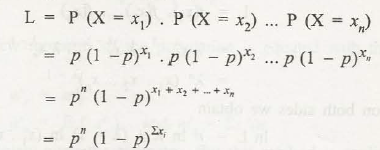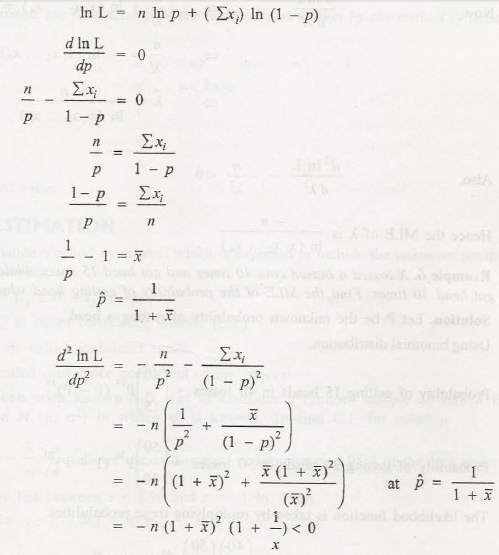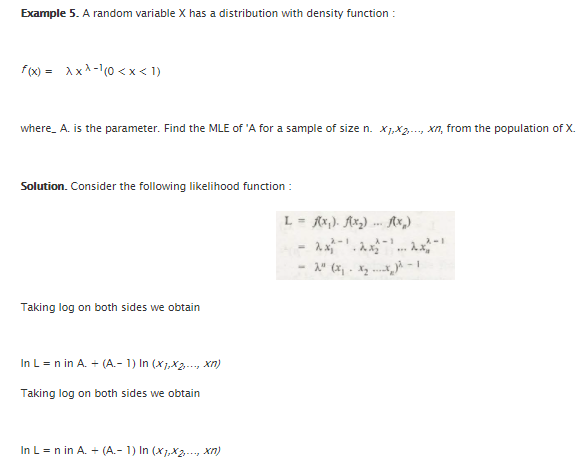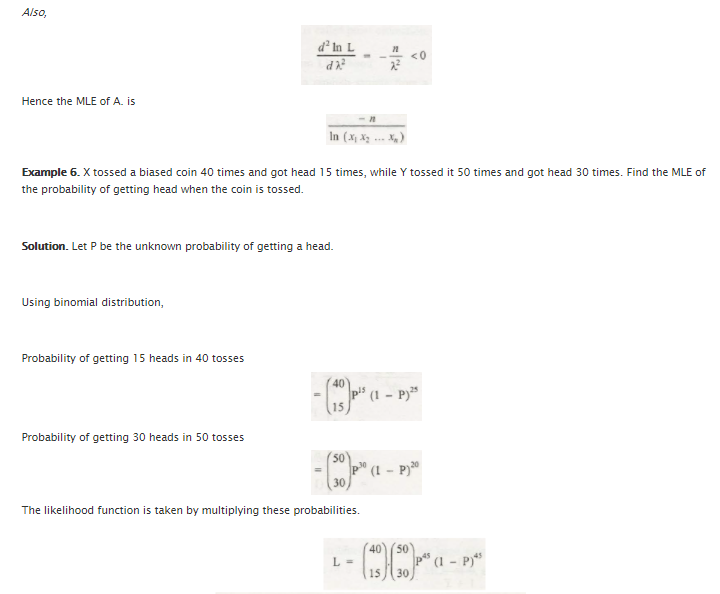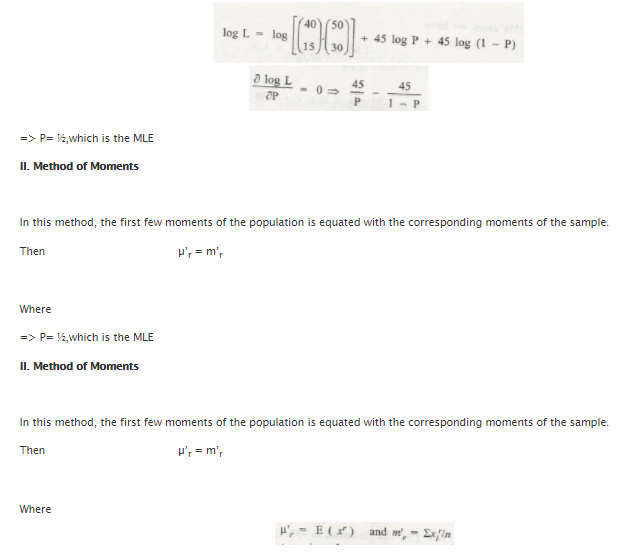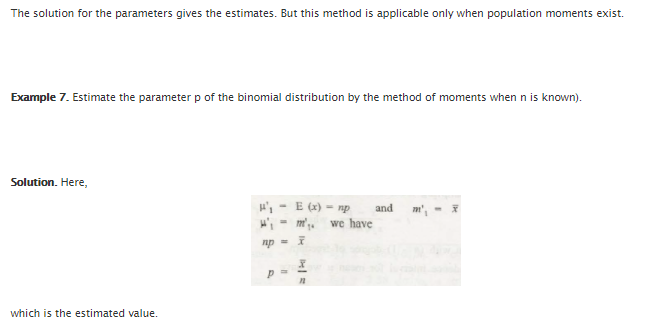### Customer Reviews

My Homework Help
Rated 5.0 out of 5 based on 510 customer reviews at